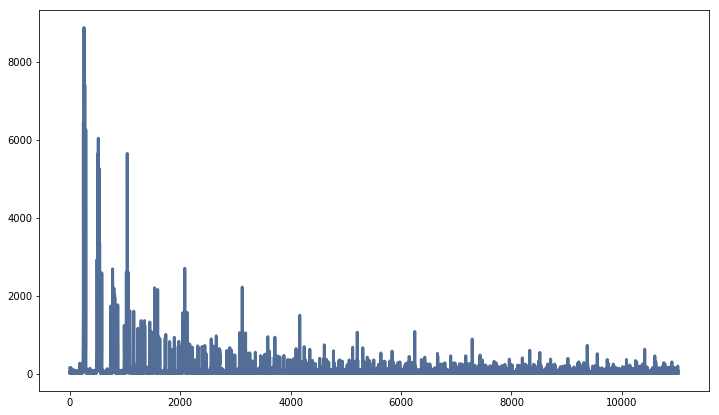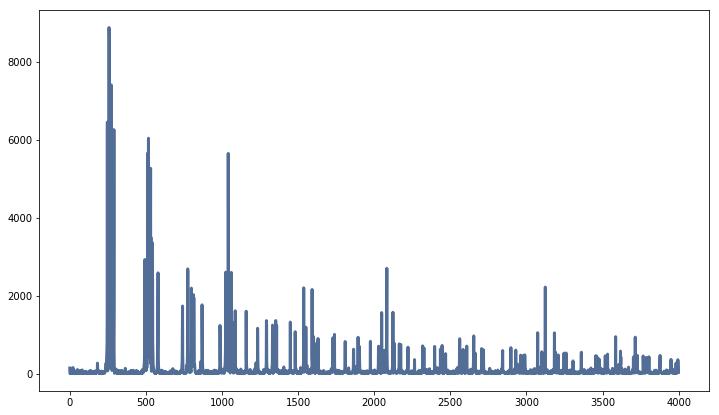Co-founder @ RMOTR

# Cacophony for the Whole Family

Allen Downey takes on elementary school band camp in this fun application of Digital Signal Processing.

Last updated: November 6th, 2018

This is an adaptation of Allen Downey's original work (see original) in O'Reilly, for the Think DSP book. The source code is published on Github.

This is an example that demonstrates some of the features in the Think DSP library.

It is inspired by the performance of a grade school band I witnessed recently. My goal is to simulate the sound of a beginner band.

In :
import thinkdsp
import thinkplot
from functools import reduce

import random

import matplotlib.pyplot as plt
%matplotlib inline


First, a function that translates from a MIDI number to a frequency:

In :
def midi_to_freq(midi_num):
"""Converts MIDI note number to frequency.

midi_num: int MIDI note number

returns: float frequency in Hz
"""
x = (midi_num - 69) / 12.0
freq = 440.0 * 2**x
return freq


Now here's a randomized version that simulates three kinds of errors: poor tuning, playing the wrong note, and popping an overtone. Notice that it is possible to make all three errors.

In :
def random_freq(midi_num):

# simulate poor tuning by adding gaussian noise to the MIDI number
midi_num += random.gauss(0, 0.5)

# one kid out of 10 plays the wrong note
if random.random() < 0.1:
midi_num += random.randint(-5, 5)

freq = midi_to_freq(midi_num)

# and one kid in 10 pops an overtone
if random.random() < 0.1:
freq *= random.randint(2, 5)

return freq


This function takes a MIDI number and duration and makes a Wave:

In :
def make_note(midi_num, duration, framerate=22050):
"""Make a MIDI note with the given duration.

midi_num: int MIDI note number
duration: float seconds
sig_cons: Signal constructor function
framerate: int frames per second

returns: Wave
"""
freq = random_freq(midi_num)
signal = thinkdsp.SawtoothSignal(freq)
wave = signal.make_wave(duration, framerate=framerate)
wave.apodize()
return wave


Let's test make_note. MIDI number 60 is middle C.

In :
note = make_note(60, 1.0)
note.make_audio()

Out:

Sounds good.

Now we can make 10 notes and play them at the same time. Since Wave provides __add__, we can use sum:

In :
def make_ensemble(midi_num, duration):
notes = [make_note(midi_num, duration) for i in range(10)]
ensemble = sum(notes)
ensemble.make_audio()
return ensemble


And we can test it with a middle C:

In :
c = make_ensemble(60, 1.0)
c.make_audio()

Out:

Good, sounds like a nest of angry wasps.

And now, a rousing chorus of that old crowd favorite, Hot Cross Buns.

Wave provides __or__, which concatenates notes, so we can use reduce:

In :
midi_nums = [64, 62, 60,     64, 62, 60,    60, 60, 60, 60,      62, 62, 62, 62,      64, 62, 60]
durations = [0.5, 0.5, 1.0,  0.5, 0.5, 1.0, 0.25, 0.25, 0.25, 0.25,  0.25, 0.25, 0.25, 0.25,  0.5, 0.5, 1.0]

In :
waves = [make_ensemble(midi_num, duration) for midi_num, duration in zip(midi_nums, durations)]
wave = reduce(thinkdsp.Wave.__or__, waves)
wave.make_audio()

Out:

And that brings a tear of pride to any parent's eye.

On a more serious note, this example tells us something about how the ear interprets complex sounds with many tones and harmonics.

Let's take a look at the spectrum of that middle C:

In :
plt.figure(figsize=(12, 7))
spectrum = c.make_spectrum()
spectrum.plot()And we can zoom in on the first few harmonics:

In :
plt.figure(figsize=(12, 7))
spectrum.plot(high=4000)A few observations:

1. The kids playing out of tune have a bigger effect on the harmonics, and less effect on the fundamental, so the ear can still pick out a clear pitch, and
2. some of the unintentional overtones overlap with the harmonics, so they change the timbre, but don't stick out as much as you might expect,
3. the high harmonics are so spread out that they basically contribute white noise and don't affect the perceived pitch.